There's one more biggie.

Remember that theorem about real zeros?

 An nth degree polynomial has, at most, n real zeros.

Well, dude, now that we have complex zeros, we can say this:

 An nth degree polynomial hasEXACTLY  n  ZEROS!*

*This includes real and complex.

So, now, if we have a 3rd degree polynomial...  we WILL get 3 zeros!

Check it out:

Find the zeros of:then draw a rough sketch of the graph:

What's his basic shape?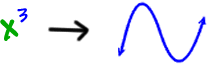Let's find those zeros: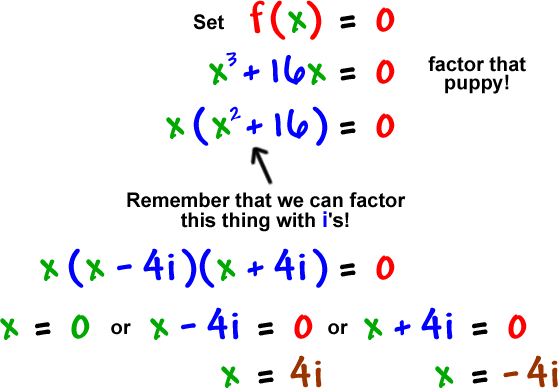zeros: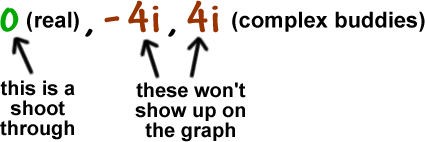The best we can do here is to make our best guess at the graph based on what we have...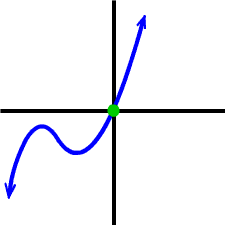or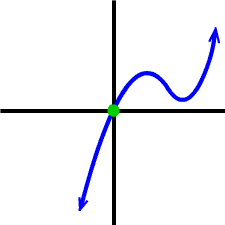Check it on the graphing calculator!  (You probably won't see any wobble at all.)